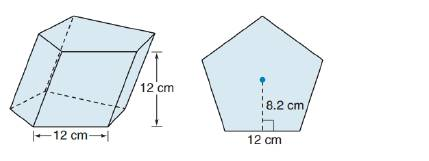Chapter 9.1, Problem 46EElementary Geometry For College St...

7th Edition
Alexander + 2 others
ISBN: 9781337614085

Solutions

Chapter
SectionElementary Geometry For College St...

7th Edition
Alexander + 2 others
ISBN: 9781337614085
Textbook Problem

For Exercise 45 to 47, consider the oblique regular pentagonal prism shown. Each side of the base measures 12 cm, and the altitude measures 12 cm.Find the total area of the prism.To determine

To find:

The total area of the prism.

Explanation

The total area of any solid is the area of all the number of lateral sides that it have, including the area of the top and the bottom of solid.

Thus, the total area (T) of any solid prism with its lateral area (L) and base area (B) is given by the formula.

T=L+2B

Here, we have 2B since, any solid prison will have two bases (One upper part and another the lower part).

Calculation:

Given,

The total are of the any prism with lateral area L and base area B is given by

T=L+2B

First let us calculate the lateral area o f the prism.

The lateral area of any prism of altitude ‘h’ and of base perimeter P is given by

L=hP

Here, we have

h=12 cm.

But, the perimeter ‘P’ of the regular pentagonal is given by the product of the measure as the base edge with 5 since, a regular pentagonal has 5 edges of equal measure.

Thus,

P = Measure of an edge × 5

=12×5

P = 60 cm

Thus, the lateral area of the parallelogram is given by

L=Ph

=6012

L=720 cm

Now, calculate the base area of the prism.

The base of the prism is a regular polygon (pentagon) with apothem 8.2 cm and of side (edge) is 12 cm

Still sussing out bartleby?

Check out a sample textbook solution.

See a sample solution

The Solution to Your Study Problems

Bartleby provides explanations to thousands of textbook problems written by our experts, many with advanced degrees!

Get Started